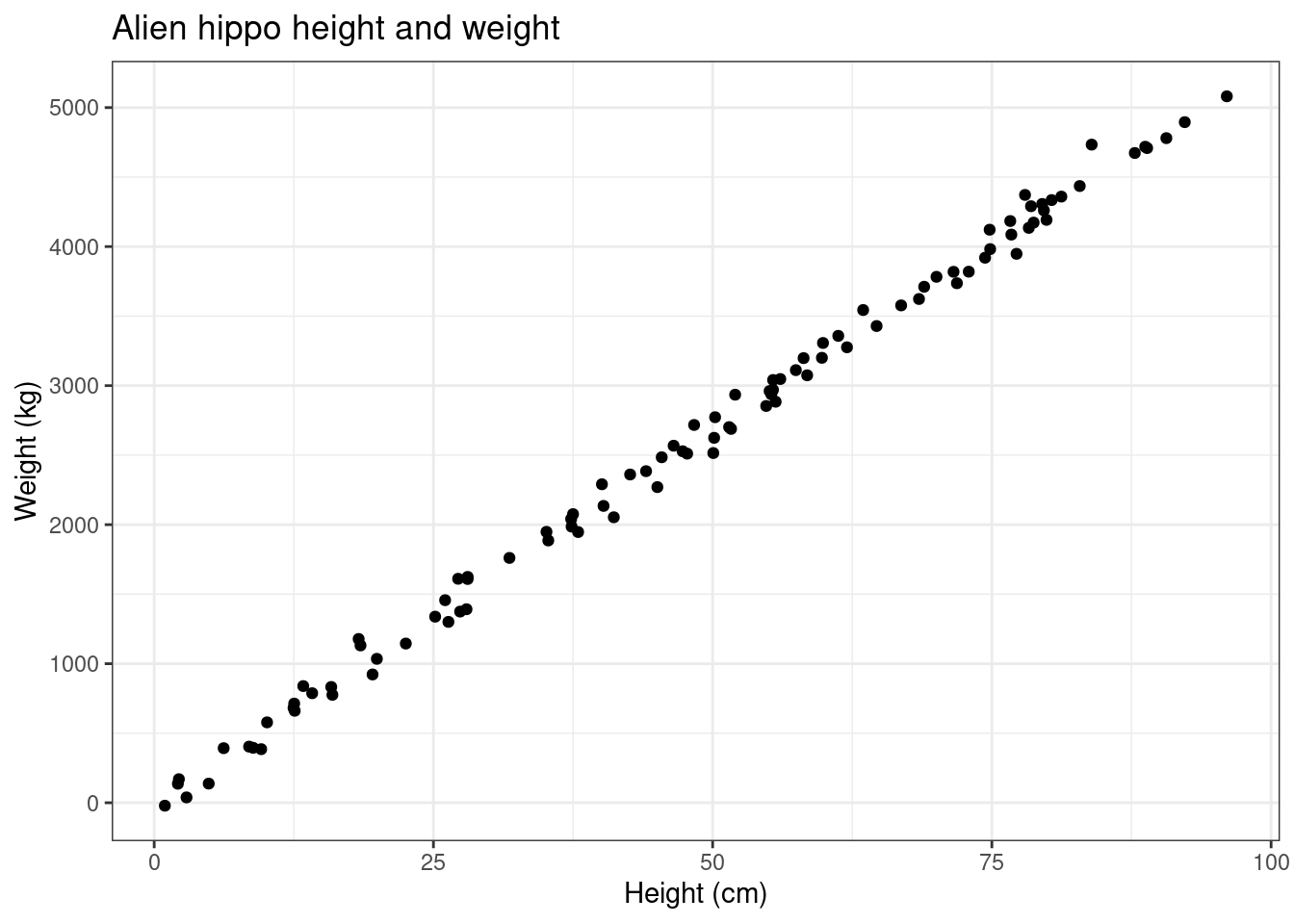17 Jul 2022

# Linear Regression Explained (Using R)Linear Regression Explained (Using R)

# Introduction

I made this blog post to help introduce what linear regression is, why it’s useful, and how it works.

We’re going to see if we can find a relationship between the heights and weights of alien hippos on Mars.

There is a youtube video that I made in conjunction with this that you should also watch if you want!

# Getting Things Set Up

Before we start any of the fun stuff, we need to get R set up!

We’re going to be making use of a library called tidyverse, a collection of packages that are useful for data science.

To install tidyverse we can run the command below. Note that we only need to run this if we haven’t installed tidyverse before.

install.packages("tidyverse")

This installs the package, but we can’t use it unless we load it up!

library(tidyverse)

Our data subjects are alien hippos on Mars.

As I mentioned previously, we’re interested to see if there is a relationship between their heights and weights.

To this end, I set sail to Mars, and recorded the heights and weights of 100 Martian hippos.

This data is convinantly stored in a CSV file online so that you don’t have to go to Mars to follow along. You can access the data like so, where we store it in a variable df.

df <- read_csv("https://raw.githubusercontent.com/curtis-murray/Teaching/main/LinearRegression/hippos.csv")

The <- symbol is an assignment operator, which is just a fancy way of saying it’s the thing that lets you assign something to something. In this case, we’re assigning the variable name df with the data read in from the CSV file (read_csv) at the specified URL.

We can view df by calling it.

df
## # A tibble: 100 × 2
##    weight height
##     <dbl>  <dbl>
##  1 3920.  74.4
##  2  385.   9.58
##  3  -21.2  0.953
##  4 1178.  18.3
##  5  833.  15.9
##  6 2485.  45.4
##  7 2854.  54.8
##  8 3820.  72.9
##  9  713.  12.5
## 10 4360.  81.2
## # … with 90 more rows

The output is a tibble with 100 rows and 2 columns. Each row corresponds to an individual Martian hippo, and the columns contain observation of their heights and weights.

# Visualisation

Visualising the relationship between height and weight is an important first step in understanding their relationship.

To do this, we can make a graph. We will use the package ggplot2 to show a plot of the hippos’ heights on the x-axis and weights on the y-axis.

df %>%
ggplot() +                                  # Create a ggplot
geom_point(aes(x = height, y = weight)) +   # Add points for height (x-axis) and weight (y-axis)
theme_bw() +                                # Styling
labs(x = "Height (cm)", y = "Weight (kg)",  # Labelling
title = "Alien hippo height and weight")Each point on this graph corresponds to a hippo. We can see that there is some relationship between the height and weight of hippos. As the height increases, so too does the weight! How can we describe this relationship?

If we could find a function $$f$$ such that, $w = f(h),$ where $$w$$ is weight, and $$h$$ is height, then we could mathematically describe the relationship between height and weight!

Okay, but how do we find this function $$f$$?

Well, here it looks like the points form a straight line that passes through the origin.

This kind of relationship is quite simple, and can be formulated as;

$\text{weight} \approx a \times \text{height},$ where $$a$$ is a variable called the height coefficient. This wiggly equals sign $$\approx$$ means “is approximately equal to”.

We don’t know what $$a$$ is yet, but once we find it we will have a mathematical formulation for weight in terms of height.

To start, let’s do a bit of guesswork to work out what $$a$$ should be.

# Trial and Error

Instead of completely blindly guessing what $$a$$ should be, let’s make a slightly informed guess. We can see that when a hippo’s height is approximately $$100$$ cm, its weight is approximately $$5000$$ kg.

Since we’re assuming $$0$$ cm tall hippos are weightless, this means that for every centimetre increase in height, there is an estimated 50kg increase in weight. Then, our height coefficient $$a$$ should be somewhere around $$50$$.

$\text{weight} \approx 50 \times \text{height}.$

Let’s have a look at what it looks like for $$a$$ between $$40$$ and $$70$$. We can change the value of $$a$$ using the slider.

We can see that when $$a=40$$, most of the points are above our line, and hence our relationship underestimates the weight. If we slide $$a$$ up to $$70$$ we now have the opposite problem! Our guess of $$a=50$$ is a lot closer, but we can do better! Try out the slider, and see if you can find a value of $$a$$ that makes the line fit best.

Okay, now that you’ve done this; what value of $$a$$ did you find to make the line fit best? More importantly; how did you work out what it meant for the line to fit best?

We need some kind of metric that tells us how well the line fits. Actually, it turns out to be a little easier to define how badly the line fits; let’s do that!

First, we can find how badly the relationship or model fits the data.

We can look at what the model would estimate/predict the weights to be, and compare these estimates/predictions to their corresponding true weights. If we find the differences between the predictions and observations, we find the errors, i.e. how bad the model is at each point.